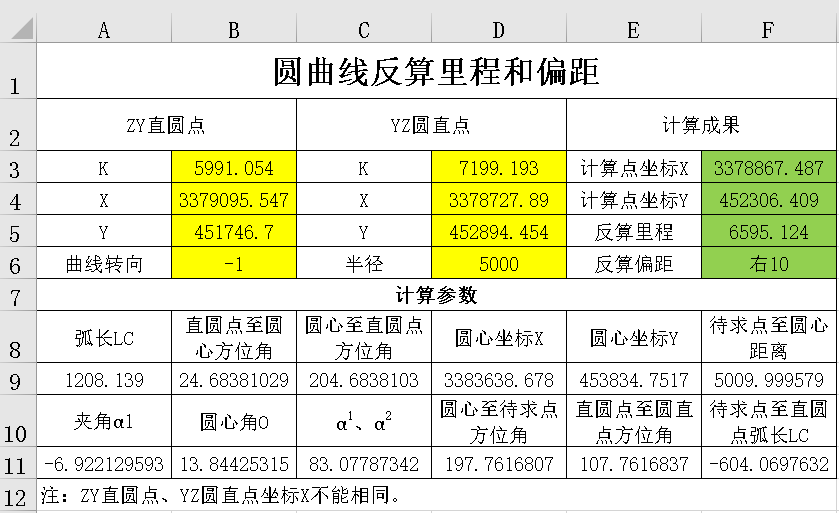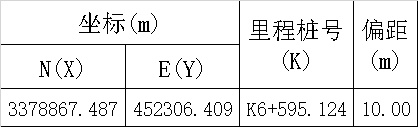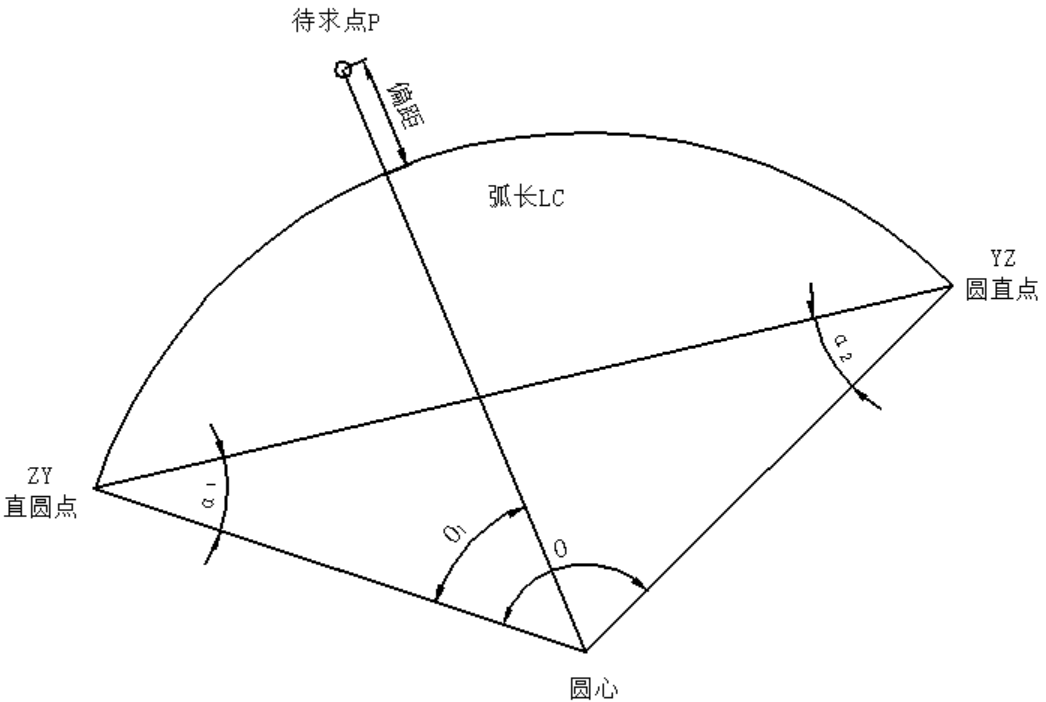1、运行Office Excel新建工作表，建立相关单元格，图示如下：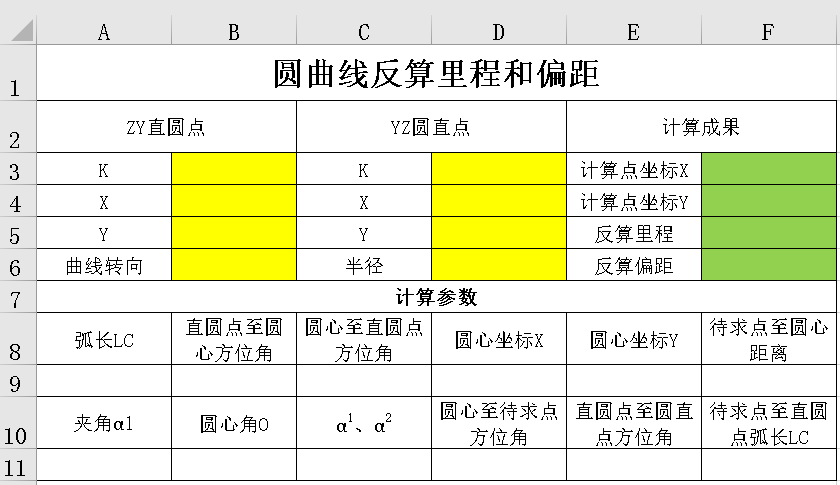2、在添加底色单元格中填写已知数据：

B3单元格输入=5991.054

B4单元格输入=3379095.547

B5单元格输入=451746.7

B6单元格输入=-1

D3单元格输入=7199.193

D4单元格输入=3378727.89

D5单元格输入=452894.454

D6单元格输入=5000

F3单元格输入=3378867.487

F4单元格输入=452306.409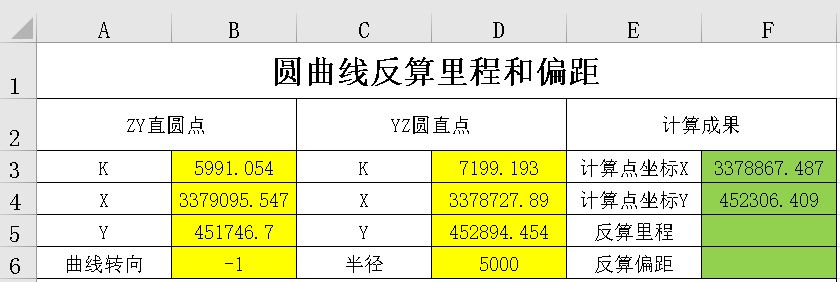3、在“计算参数”区域填入以下公式：

A9单元格输入=D3-B3

B9单元格输入=IF(B6）0,E11+C11,E11-C11)

C9单元格输入=IF(B9）180,B9-180,B9+180)

D9单元格输入=B4+D6*COS(B9*PI()/180)

E9单元格输入=B5+D6*SIN(B9*PI()/180)

F9单元格输入=SQRT((F4-E9)^2+(F3-D9)^2)

A11单元格输入=D11-C9

B11单元格输入=A9*180/PI()/D6

C11单元格输入=(180-B11)/2

D11单元格输入=IF(AND(F4-E9）0,F3-D9）0),ATAN((F4-E9)/(F3-D9))*180/PI(),IF(AND(F3-D9（0,F4-E9）0),ATAN((F4-E9)/(F3-D9))*180/PI()+180,IF(AND(F4-E9（0,F3-D9（0),ATAN((F4-E9)/(F3-D9))*180/PI()+180,ATAN((F4-E9)/(F3-D9))*180/PI()+360)))

E11单元格输入=IF(AND(D5-B5）0,D4-B4）0),ATAN((D5-B5)/(D4-B4))*180/PI(),IF(AND(D4-B4（0,D5-B5）0),ATAN((D5-B5)/(D4-B4))*180/PI()+180,IF(AND(D5-B5（0,D4-B4（0),ATAN((D5-B5)/(D4-B4))*180/PI()+180,ATAN((D5-B5)/(D4-B4))*180/PI()+360)))

F11单元格输入=A11*PI()*D6/180

F5单元格输入=B3+ABS(F11)

F6单元格输入=IF(F9（D6,”左”,IF(F9）D6,”右”,”中”))&ROUND(ABS(F9-D6),3)